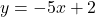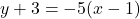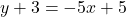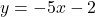Y+3= -5(x-1) write the equation in slope intercept form￼

Question

Y+3= -5(x-1) write the equation in slope intercept form￼

in progress 0
5 months 2021-08-22T11:33:58+00:00 1 Answers 4 views 0

1. Answer: The equation in slope-intercept form:Step-by-step explanation:

The given equation of a line :The equation of a line in slope -intercept form: y=mx+c, where m= slope , c=y-intercept.

We first use distributive property(a(b+c)=ab+ac) to simplify the given equation, we getSubtract 3 from both sides, we getHence, the equation in slope-intercept form: